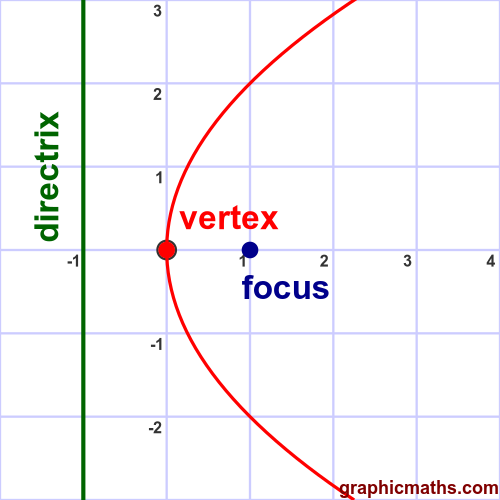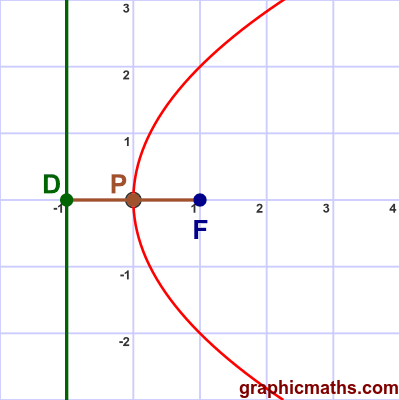# Parabola focus and directrix

Martin McBride, 2020-09-13
Tags parabola locus focus directrix
Categories coordinate systems pure mathematics

We have previously seen how a parabola is defined in terms of parametric equations or alternatively in Cartesian form.

An alternative way to define a parabola is as a locus of points.

## Focus and directrix

The locus defining a parabola depends on a focus and a directrix.

The focus is a point. For a standard parabola, the focus is located on the x axis a distance $a$ from the origin, that is at the point (a, 0). $a$ is the constant in the parabola equation $y^2 = 4 a x$

The directrix is a line. For a standard parabola it is a line perpendicular to the x axis passing through (-a, 0), that is the line $x = -a$

The vertex of the parabola is the turning point. It is always halfway between the focus and directrix, which is always at the origin for a standard parabola.

This diagram shows the focus, directrix and vertex for the case $a = 1$:## The locus

A parabola is the locus of all points which are an equal distance from the focus and directrix:

$$FP = PD$$

The animation below illustrates this: